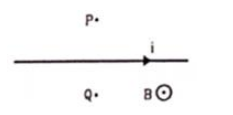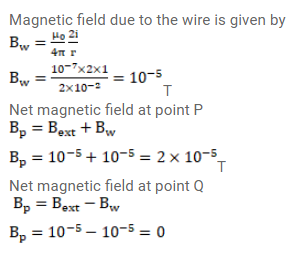# A long, straight wire carrying a current of

Question:

A long, straight wire carrying a current of $1.0 \mathrm{~A}$ is placed horizontally in a uniform magnetic field $\mathrm{B}=1.0 \times 10^{-5} \mathrm{~T}$ pointing vertically upward. Find the magnitude of the resultant magnetic field at the points $P$ and $Q$, both situated at a distance of $2.0 \mathrm{~cm}$ from the wire in the same horizontal plane.Solution: